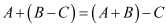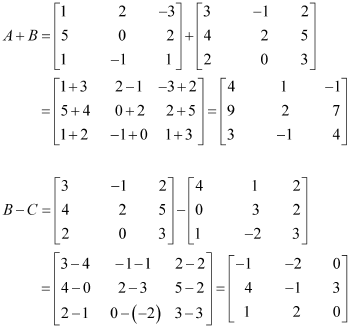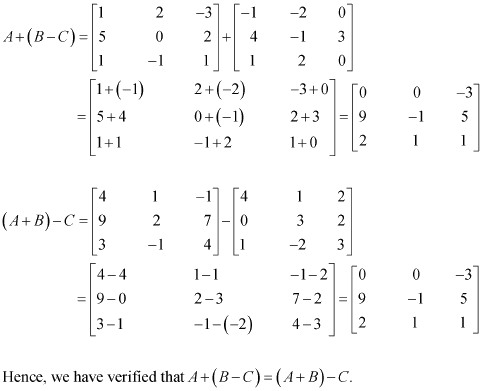# 3 – MATRICES

Here we are going through the ncert solutions for class 12 maths chapter 3 – matrices so before going through the ncert solutions make sure to go through the textbook that helps you to understand the solutions more easily

#### Question 1:

In the matrix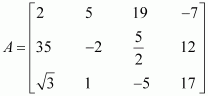, write:

(i) The order of the matrix (ii) The number of elements,

(iii) Write the elements a13a21a33a24a23

(i) In the given matrix, the number of rows is 3 and the number of columns is 4. Therefore, the order of the matrix is 3 × 4.

(ii) Since the order of the matrix is 3 × 4, there are 3 × 4 = 12 elements in it.

(iii) a13 = 19, a21 = 35, a33 = −5, a24 = 12, a23 =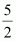#### Question 2:

If a matrix has 24 elements, what are the possible order it can have? What, if it has 13 elements?

We know that if a matrix is of the order m × n, it has mn elements. Thus, to find all the possible orders of a matrix having 24 elements, we have to find all the ordered pairs of natural numbers whose product is 24.

The ordered pairs are: (1, 24), (24, 1), (2, 12), (12, 2), (3, 8), (8, 3), (4, 6), and
(6, 4)

Hence, the possible orders of a matrix having 24 elements are:

1 × 24, 24 × 1, 2 × 12, 12 × 2, 3 × 8, 8 × 3, 4 × 6, and 6 × 4

(1, 13) and (13, 1) are the ordered pairs of natural numbers whose product is 13.

Hence, the possible orders of a matrix having 13 elements are 1 × 13 and 13 × 1.

#### Question 3:

If a matrix has 18 elements, what are the possible orders it can have? What, if it has 5 elements?

We know that if a matrix is of the order m × n, it has mn elements. Thus, to find all the possible orders of a matrix having 18 elements, we have to find all the ordered pairs of natural numbers whose product is 18.

The ordered pairs are: (1, 18), (18, 1), (2, 9), (9, 2), (3, 6,), and (6, 3)

Hence, the possible orders of a matrix having 18 elements are:

1 × 18, 18 × 1, 2 × 9, 9 × 2, 3 × 6, and 6 × 3

(1, 5) and (5, 1) are the ordered pairs of natural numbers whose product is 5.

Hence, the possible orders of a matrix having 5 elements are 1 × 5 and 5 × 1.

#### Question 4:

Construct a 2 × 2 matrix,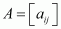, whose elements are given by:

(i)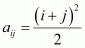(ii)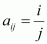(iii)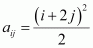In general, a 2 × 2 matrix is given by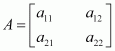(i)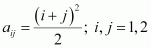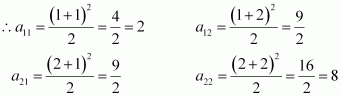Therefore, the required matrix is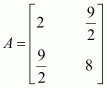(ii)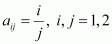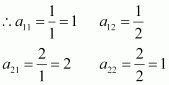Therefore, the required matrix is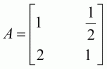(iii)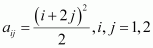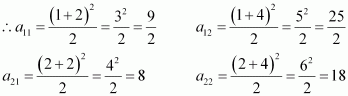Therefore, the required matrix is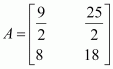#### Question 5:

Construct a 3 × 4 matrix, whose elements are given by

(i)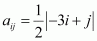(ii)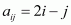In general, a 3 × 4 matrix is given by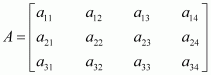(i)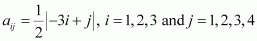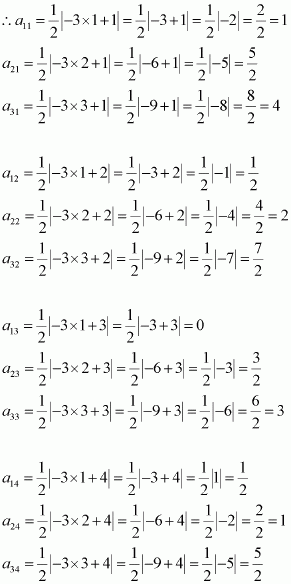Therefore, the required matrix is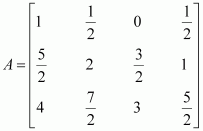(ii)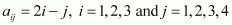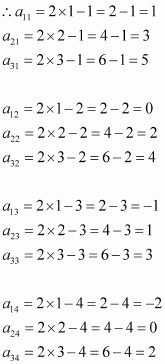Therefore, the required matrix is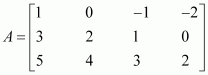#### Question 6:

Find the value of xy, and z from the following equation:

(i)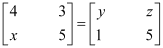(ii)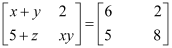(iii)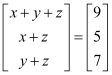(i)As the given matrices are equal, their corresponding elements are also equal.

Comparing the corresponding elements, we get:

x = 1, y = 4, and z = 3

(ii)As the given matrices are equal, their corresponding elements are also equal.

Comparing the corresponding elements, we get:

x + y = 6, xy = 8, 5 + = 5

Now, 5 + z = 5  z = 0

We know that:

(x − y)2 = (x + y)2 − 4xy

(x − y)2 = 36 − 32 = 4

x − y = ±2

Now, when x − y = 2 and x + y = 6, we get x = 4 and y = 2

When x − = − 2 and x + y = 6, we get x = 2 and = 4

x = 4, y = 2, and z = 0 or x = 2, y = 4, and z = 0

(iii)As the two matrices are equal, their corresponding elements are also equal.

Comparing the corresponding elements, we get:

x + y + z = 9 … (1)

x + z = 5 … (2)

y + z = 7 … (3)

From (1) and (2), we have:

+ 5 = 9

y = 4

Then, from (3), we have:

4 + z = 7

z = 3

x + z = 5

x = 2

x = 2, y = 4, and z = 3

#### Question 7:

Find the value of abc, and d from the equation: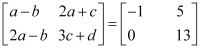As the two matrices are equal, their corresponding elements are also equal.

Comparing the corresponding elements, we get:

a − b = −1 … (1)

2a − b = 0 … (2)

2a + c = 5 … (3)

3c + d = 13 … (4)

From (2), we have:

b = 2a

Then, from (1), we have:

− 2a = −1

a = 1

b = 2

Now, from (3), we have:

2 ×1 + c = 5

c = 3

From (4) we have:

3 ×3 + d = 13

9 + = 13  d = 4

a = 1, b = 2, c = 3, and d = 4

#### Question 8: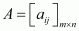is a square matrix, if

(A) m < n

(B) m > n

(C) m = n

(D) None of these

It is known that a given matrix is said to be a square matrix if the number of rows is equal to the number of columns.

Therefore,is a square matrix, if m = n.

#### Question 9:

Which of the given values of x and y make the following pair of matrices equal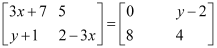(A)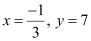(B) Not possible to find

(C)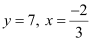(D)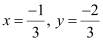It is given thatEquating the corresponding elements, we get: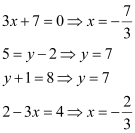We find that on comparing the corresponding elements of the two matrices, we get two different values of x, which is not possible.

Hence, it is not possible to find the values of x and y for which the given matrices are equal.

#### Question 10:

The number of all possible matrices of order 3 × 3 with each entry 0 or 1 is:

(A) 27

(B) 18

(C) 81

(D) 512

The given matrix of the order 3 × 3 has 9 elements and each of these elements can be either 0 or 1.

Now, each of the 9 elements can be filled in two possible ways.

Therefore, by the multiplication principle, the required number of possible matrices is 29 = 512

#### Question 1:

Let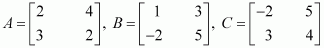Find each of the following

(i)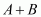(ii)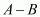(iii)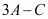(iv)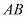(v)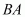(i)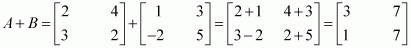(ii)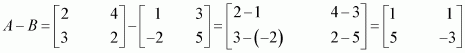(iii)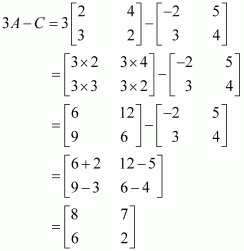(iv) Matrix A has 2 columns. This number is equal to the number of rows in matrix B. Therefore, AB is defined as: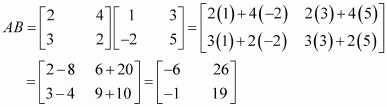(v) Matrix B has 2 columns. This number is equal to the number of rows in matrix A. Therefore, BA is defined as: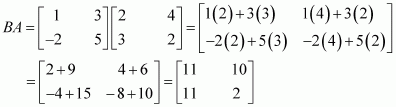#### Question 2:

Compute the following:

(i)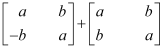(ii)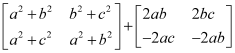(iii)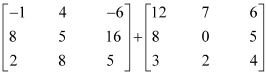(v)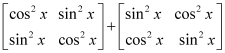(i)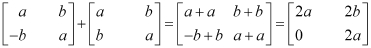(ii)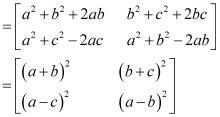(iii)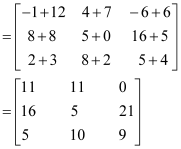(iv)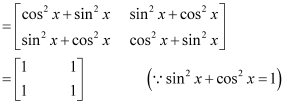#### Question 3:

Compute the indicated products

(i)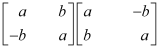(ii)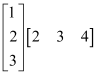(iii)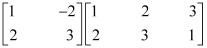(iv)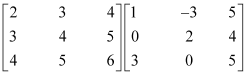(v)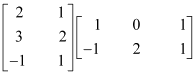(vi)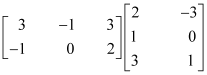(i)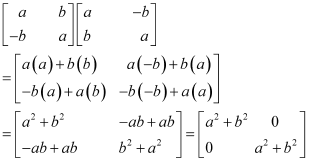(ii)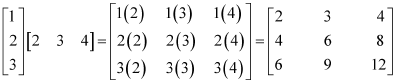(iii)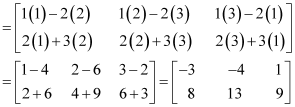(iv)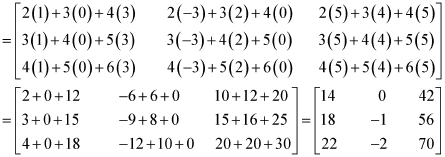(v)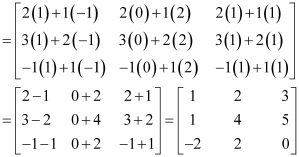(vi)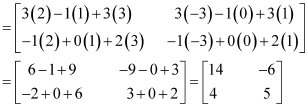#### ​​ Question 4:

If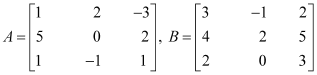, and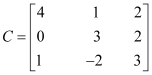, then compute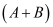and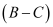. Also, verify that